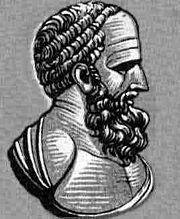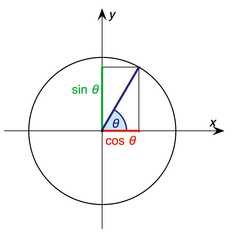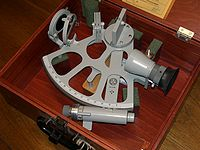# Trigonometry

﻿
TrigonometryThe Canadarm2 robotic manipulator on the International Space Station is operated by controlling the angles of its joints. Calculating the final position of the astronaut at the end of the arm requires repeated use of trigonometric functions of those angles.

Trigonometry (from Greek trigōnon "triangle" + metron "measure") is a branch of mathematics that studies triangles and the relationships between their sides and the angles between these sides. Trigonometry defines the trigonometric functions, which describe those relationships and have applicability to cyclical phenomena, such as waves. The field evolved during the third century BC as a branch of geometry used extensively for astronomical studies. It is also the foundation of the practical art of surveying.

Trigonometry basics are often taught in middle school or junior high school as part of the basic math curriculum. More in depth trigonometry is sometimes taught in high school either as a separate course or as part of a precalculus course. The trigonometric functions are pervasive in parts of pure mathematics and applied mathematics such as Fourier analysis and the wave equation, which are in turn essential to many branches of science and technology. Spherical trigonometry studies triangles on spheres, surfaces of constant positive curvature, in elliptic geometry. It is fundamental to astronomy and navigation. Trigonometry on surfaces of negative curvature is part of Hyperbolic geometry.

## HistoryThe first trigonometric table was apparently compiled by Hipparchus, who is now consequently known as "the father of trigonometry."

Sumerian astronomers introduced angle measure, using a division of circles into 360 degrees. They and their successors the Babylonians studied the ratios of the sides of similar triangles and discovered some properties of these ratios, but did not turn that into a systematic method for finding sides and angles of triangles. The ancient Nubians used a similar methodology. The ancient Greeks transformed trigonometry into an ordered science.

Classical Greek mathematicians (such as Euclid and Archimedes) studied the properties of chords and inscribed angles in circles, and proved theorems that are equivalent to modern trigonometric formulae, although they presented them geometrically rather than algebraically. Claudius Ptolemy expanded upon Hipparchus' Chords in a Circle in his Almagest. The modern sine function was first defined in the Surya Siddhanta, and its properties were further documented by the 5th century Indian mathematician and astronomer Aryabhata. These Greek and Indian works were translated and expanded by medieval Islamic mathematicians. By the 10th century, Islamic mathematicians were using all six trigonometric functions, had tabulated their values, and were applying them to problems in spherical geometry.[citation needed] At about the same time, Chinese mathematicians developed trigonometry independently, although it was not a major field of study for them. Knowledge of trigonometric functions and methods reached Europe via Latin translations of the works of Persian and Arabic astronomers such as Al Battani and Nasir al-Din al-Tusi. One of the earliest works on trigonometry by a European mathematician is De Triangulis by the 15th century German mathematician Regiomontanus. Trigonometry was still so little known in 16th century Europe that Nicolaus Copernicus devoted two chapters of De revolutionibus orbium coelestium to explaining its basic concepts.

Driven by the demands of navigation and the growing need for accurate maps of large areas, trigonometry grew to be a major branch of mathematics. Bartholomaeus Pitiscus was the first to use the word, publishing his Trigonometria in 1595. Gemma Frisius described for the first time the method of triangulation still used today in surveying. It was Leonhard Euler who fully incorporated complex numbers into trigonometry. The works of James Gregory in the 17th century and Colin Maclaurin in the 18th century were influential in the development of trigonometric series. Also in the 18th century, Brook Taylor defined the general Taylor series.

## OverviewIn this right triangle: sin A = a/c; cos A = b/c; tan A = a/b.

If one angle of a triangle is 90 degrees and one of the other angles is known, the third is thereby fixed, because the three angles of any triangle add up to 180 degrees. The two acute angles therefore add up to 90 degrees: they are complementary angles. The shape of a triangle is completely determined, except for similarity, by the angles. Once the angles are known, the ratios of the sides are determined, regardless of the overall size of the triangle. If the length of one of the sides is known, the other two are determined. These ratios are given by the following trigonometric functions of the known angle A, where a, b and c refer to the lengths of the sides in the accompanying figure:

• Sine function (sin), defined as the ratio of the side opposite the angle to the hypotenuse.$\sin A=\frac{\textrm{opposite}}{\textrm{hypotenuse}}=\frac{a}{\,c\,}\,.$
• Cosine function (cos), defined as the ratio of the adjacent leg to the hypotenuse.$\cos A=\frac{\textrm{adjacent}}{\textrm{hypotenuse}}=\frac{b}{\,c\,}\,.$
• Tangent function (tan), defined as the ratio of the opposite leg to the adjacent leg.$\tan A=\frac{\textrm{opposite}}{\textrm{adjacent}}=\frac{a}{\,b\,}=\frac{\sin A}{\cos A}\,.$

The hypotenuse is the side opposite to the 90 degree angle in a right triangle; it is the longest side of the triangle, and one of the two sides adjacent to angle A. The adjacent leg is the other side that is adjacent to angle A. The opposite side is the side that is opposite to angle A. The terms perpendicular and base are sometimes used for the opposite and adjacent sides respectively. Many English speakers find it easy to remember what sides of the right triangle are equal to sine, cosine, or tangent, by memorizing the word SOH-CAH-TOA (see below under Mnemonics).

The reciprocals of these functions are named the cosecant (csc or cosec), secant (sec), and cotangent (cot), respectively. The inverse functions are called the arcsine, arccosine, and arctangent, respectively. There are arithmetic relations between these functions, which are known as trigonometric identities. The cosine, cotangent, and cosecant are so named because they are respectively the sine, tangent, and secant of the complementary angle abbreviated to "co-".

With these functions one can answer virtually all questions about arbitrary triangles by using the law of sines and the law of cosines. These laws can be used to compute the remaining angles and sides of any triangle as soon as two sides and their included angle or two angles and a side or three sides are known. These laws are useful in all branches of geometry, since every polygon may be described as a finite combination of triangles.

### Extending the definitionsFig. 1a - Sine and cosine of an angle θ defined using the unit circle.

The above definitions apply to angles between 0 and 90 degrees (0 and π/2 radians) only. Using the unit circle, one can extend them to all positive and negative arguments (see trigonometric function). The trigonometric functions are periodic, with a period of 360 degrees or 2π radians. That means their values repeat at those intervals. The tangent and cotangent functions also have a shorter period, of 180 degrees or π radians.

The trigonometric functions can be defined in other ways besides the geometrical definitions above, using tools from calculus and infinite series. With these definitions the trigonometric functions can be defined for complex numbers. The complex exponential function is particularly useful.

ex + iy = ex(cos y + isin y).

See Euler's and De Moivre's formulas.

### Mnemonics

A common use of mnemonics is to remember facts and relationships in trigonometry. For example, the sine, cosine, and tangent ratios in a right triangle can be remembered by representing them as strings of letters. For instance, a mnemonic for English speakers is SOH-CAH-TOA:

Sine = Opposite ÷ Hypotenuse

One way to remember the letters is to sound them out phonetically (i.e. "SOH-CAH-TOA", which is pronounced 'so-kə-tow'-uh'). Another method is to expand the letters into a sentence, such as "Some Old Hippy Caught Another Hippy Trippin' On Acid".

### Calculating trigonometric functions

Trigonometric functions were among the earliest uses for mathematical tables. Such tables were incorporated into mathematics textbooks and students were taught to look up values and how to interpolate between the values listed to get higher accuracy. Slide rules had special scales for trigonometric functions.

Today scientific calculators have buttons for calculating the main trigonometric functions (sin, cos, tan and sometimes cis) and their inverses. Most allow a choice of angle measurement methods: degrees, radians and, sometimes, grad.[citation needed] Most computer programming languages provide function libraries that include the trigonometric functions. The floating point unit hardware incorporated into the microprocessor chips used in most personal computers have built-in instructions for calculating trigonometric functions.[citation needed]

## Applications of trigonometrySextants are used to measure the angle of the sun or stars with respect to the horizon. Using trigonometry and a marine chronometer, the position of the ship can be determined from such measurements.

There are an enormous number of uses of trigonometry and trigonometric functions. For instance, the technique of triangulation is used in astronomy to measure the distance to nearby stars, in geography to measure distances between landmarks, and in satellite navigation systems. The sine and cosine functions are fundamental to the theory of periodic functions such as those that describe sound and light waves.

Fields that use trigonometry or trigonometric functions include astronomy (especially for locating apparent positions of celestial objects, in which spherical trigonometry is essential) and hence navigation (on the oceans, in aircraft, and in space), music theory, acoustics, optics, analysis of financial markets, electronics, probability theory, statistics, biology, medical imaging (CAT scans and ultrasound), pharmacy, chemistry, number theory (and hence cryptology), seismology, meteorology, oceanography, many physical sciences, land surveying and geodesy, architecture, phonetics, economics, electrical engineering, mechanical engineering, civil engineering, computer graphics, cartography, crystallography and game development.

## Standard identities

Identities are those equations that hold true for any value.$\sin^2 A + \cos^2 A = 1 \$$\sec^2 A - \tan^2 A = 1 \$$\csc^2 A - \cot^2 A = 1 \$

## Angle transformation formulae$\sin (A + B) = \sin A \cdot \cos B + \cos A \cdot \sin B$$\cos (A + B) = \cos A \cdot \cos B - \sin A \cdot \sin B$$\sin (A - B) = \sin A \cdot \cos B - \cos A \cdot \sin B$$\cos (A - B) = \cos A \cdot \cos B + \sin A \cdot \sin B$

## Common formulasTriangle with sides a,b,c and respectively opposite angles A,B,C

Certain equations involving trigonometric functions are true for all angles and are known as trigonometric identities. Some identities equate an expression to a different expression involving the same angles. These are listed in List of trigonometric identities. Triangle identities that relate the sides and angles of a given triangle are listed below.

In the following identities, A, B and C are the angles of a triangle and a, b and c are the lengths of sides of the triangle opposite the respective angles.

### Law of sines

The law of sines (also known as the "sine rule") for an arbitrary triangle states:$\frac{a}{\sin A} = \frac{b}{\sin B} = \frac{c}{\sin C} = 2R,$

where R is the radius of the circumscribed circle of the triangle:$R = \frac{abc}{\sqrt{(a+b+c)(a-b+c)(a+b-c)(b+c-a)}}.$

Another law involving sines can be used to calculate the area of a triangle. Given two sides and the angle between the sides, the area of the triangle is:$\mbox{Area} = \frac{1}{2}a b\sin C.$All of the trigonometric functions of an angle θ can be constructed geometrically in terms of a unit circle centered at O.

### Law of cosines

The law of cosines (known as the cosine formula, or the "cos rule") is an extension of the Pythagorean theorem to arbitrary triangles:$c^2=a^2+b^2-2ab\cos C ,\,$

or equivalently:$\cos C=\frac{a^2+b^2-c^2}{2ab}.\,$

### Law of tangents

The law of tangents:$\frac{a-b}{a+b}=\frac{\tan\left[\tfrac{1}{2}(A-B)\right]}{\tan\left[\tfrac{1}{2}(A+B)\right]}$

### Euler's formula

Euler's formula, which states that eix = cos x + isin x, produces the following analytical identities for sine, cosine, and tangent in terms of e and the imaginary unit i:$\sin x = \frac{e^{ix} - e^{-ix}}{2i}, \qquad \cos x = \frac{e^{ix} + e^{-ix}}{2}, \qquad \tan x = \frac{i(e^{-ix} - e^{ix})}{e^{ix} + e^{-ix}}.$

Wikimedia Foundation. 2010.

### Look at other dictionaries:

• Trigonometry — Trig o*nom e*try, n.; pl. { tries}. [Gr. ? a triangle + metry: cf. F. trigonom[ e]trie. See {Trigon}.] 1. That branch of mathematics which treats of the relations of the sides and angles of triangles, which the methods of deducing from certain… …   The Collaborative International Dictionary of English

• trigonometry — branch of mathematics that deals with relations between sides and angles of triangles, 1610s, from Mod.L. trigonometria (Barthelemi Pitiscus, 1595), from Gk. trigonon triangle (from tri three + gonia angle; see KNEE (Cf. knee)) + metron a measure …   Etymology dictionary

• trigonometry — ► NOUN ▪ the branch of mathematics concerned with the relations of the sides and angles of triangles and with the relevant functions of any angles. DERIVATIVES trigonometric adjective trigonometrical adjective. ORIGIN from Greek trig nos three… …   English terms dictionary

• trigonometry — [trig΄ə näm′ə trē] n. pl. trigonometries [ModL trigonometria < Gr trigōnon, triangle (see TRIGON) + metria, measurement (see METRY)] the branch of mathematics that deals with the ratios between the sides of a right triangle with reference to… …   English World dictionary

• trigonometry — trigonometric /trig euh neuh me trik/, trigonometrical, adj. trigonometrically, adv. /trig euh nom i tree/, n. the branch of mathematics that deals with the relations between the sides and angles of plane or spherical triangles, and the… …   Universalium

• trigonometry — Synonyms and related words: Boolean algebra, Euclidean geometry, Fourier analysis, Lagrangian function, algebra, algebraic geometry, analysis, analytic geometry, arithmetic, associative algebra, binary arithmetic, calculus, circle geometry,… …   Moby Thesaurus

• Trigonometry — Mathematics Math e*mat ics, n. [F. math[ e]matiques, pl., L. mathematica, sing., Gr. ? (sc. ?) science. See {Mathematic}, and { ics}.] That science, or class of sciences, which treats of the exact relations existing between quantities or… …   The Collaborative International Dictionary of English

• trigonometry — [[t]trɪ̱gənɒ̱mɪtri[/t]] N UNCOUNT Trigonometry is the branch of mathematics that is concerned with calculating the angles of triangles or the lengths of their sides …   English dictionary

• trigonometry — noun Etymology: New Latin trigonometria, from Greek trigōnon + metria metry Date: 1614 the study of the properties of triangles and trigonometric functions and of their applications …   New Collegiate Dictionary

• trigonometry — noun The branch of mathematics that deals with the relationships between the sides and the angles of triangles and the calculations based on them, particularly the trigonometric functions. See Also: geometry, trigon, trigonometric, trigonometrist …   Wiktionary